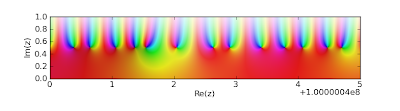# Zeta evaluation with the Riemann-Siegel expansion

January 19, 2010

I’m very grateful to Juan Arias de Reyna who has contributed a module implementing zeta function evaluation using the Riemann-Siegel formula in mpmath. This finally permits rapid evaluation of the Riemann zeta function for arguments with large imaginary part.

To follow tradition on this blog, pictorial proof shall be given. Here is a plot of a segment of the critical line, ζ(1/2+ti) with t between 1010+50 and 1010+55:A complex plot of showing the critical strip, t ranging between 108+40 and 108+45 (note: the y scale is reversed):Juan Arias de Reyna, who is a professor of mathematics at the University of Seville, has done a thorough job with this code. He has even proved rigorous error bounds for his algorithm (subject to assumptions that the underlying functions in mpmath being well-implemented). The news is that the bounds — documented in an as-yet unpublished paper — are valid off the critical line. The code also computes derivatives (up to 4th derivatives), although not as rigorously but still very robustly.

I integrated the code (and added a few optimizations) during the last few days. The zeta function in mpmath now automatically switches between Borwein’s algorithm (close to the real line), Euler-Maclaurin summation, and the Riemann-Siegel expansion.

Some example values and timings on my laptop, computing ζ(1/2+10ni) for n up to 12:

>>> from timeit import default_timer as clock
>>> mp.dps = 25
>>>
>>> for n in range(13):
...     t1 = clock(); y = zeta(0.5 + 10**n*j); t2 = clock()
...     print n, y, round(t2-t1,3)
...
0 (0.1439364270771890603243897 - 0.7220997435316730891261751j) 0.02
1 (1.544895220296752766921496 - 0.1153364652712733754365914j) 0.003
2 (2.692619885681324090476096 - 0.02038602960259816177072685j) 0.042
3 (0.3563343671943960550744025 + 0.9319978312329936651150604j) 0.073
4 (-0.3393738026388344575674711 - 0.03709150597320603147434421j) 0.434
5 (1.073032014857753132114076 + 5.780848544363503984261041j) 0.167
6 (0.07608906973822710000556456 + 2.805102101019298955393837j) 0.146
7 (11.45804061057709254500227 - 8.643437226836021723818215j) 0.181
8 (-3.362839487530727943146807 + 1.407234559646447885979583j) 0.336
9 (-2.761748029838060942376813 - 1.677512240989459839213205j) 0.877
10 (0.3568002308560733825395879 + 0.286505849095836103292093j) 2.695
11 (0.6436639255801185727194357 + 0.1168615914616853418448829j) 8.583
12 (2.877961809278403355251079 - 3.206771071318398923493412j) 26.934

Similar results off the critical line:

>>> for n in range(13):
...     t1 = clock(); y = zeta(1.0 + 10**n*j); t2 = clock()
...     print n, y, round(t2-t1,3)
...
0 (0.5821580597520036481994632 - 0.9268485643308070765364243j) 0.021
1 (1.390287313237401426796005 - 0.109785153066302056909746j) 0.004
2 (1.632833506686711866610705 - 0.06813120384181249010120548j) 0.043
3 (0.9409368682927533108010138 + 0.04522665207209509908865644j) 0.083
4 (0.4973279229716308441790286 - 0.5878238243194009766923214j) 0.598
5 (1.618122122846936796567759 + 1.070441041470623686626035j) 0.233
6 (0.9473872625104789104802422 + 0.5942199931209183283333071j) 0.195
7 (2.859846483332530337008882 + 0.491808047480981808903986j) 0.409
8 (1.83493089532784660651756 + 1.069184790423612896917448j) 0.455
9 (0.9038018561650776977609945 - 1.189857828822373901473908j) 1.393
10 (0.5418173564211820524034624 + 0.635303581895880322679247j) 3.824
11 (0.5365466615361310937110304 - 0.1234443975100346650640542j) 12.031
12 (0.8225630719679733497367277 - 0.4484762282040223492401122j) 38.061

The implementation also supports use of the fp context. Double precision unavoidably becomes insufficient as the imaginary part approaches 1015, but it has the advantage of speed in the range where it works:

>>> for n in range(13):
...     t1 = clock(); y = fp.zeta(0.5 + 10**n*1j); t2 = clock()
...     print n, y, round(t2-t1,3)
...
0 (0.143936427077-0.722099743532j) 0.007
1 (1.5448952203-0.115336465271j) 0.001
2 (2.69261988568-0.0203860296026j) 0.003
3 (0.356334367195+0.931997831233j) 0.004
4 (-0.339373802616-0.0370915059691j) 0.123
5 (1.07303201485+5.78084854433j) 0.005
6 (0.076089072636+2.80510210471j) 0.006
7 (11.4580404601-8.64343725721j) 0.006
8 (-3.36283920435+1.40723433071j) 0.011
9 (-2.76174796643-1.67750591108j) 0.028
10 (0.356829034142+0.286525774475j) 0.083
11 (0.64314751322+0.116713738713j) 0.256
12 (2.8689206645-3.21135962379j) 0.808

We have done some comparison with Mathematica, and the mpmath version appears to be about as fast (a bit faster or a bit slower, sometimes substantially faster, depending on circumstance). The most expensive part of the computation occurs in a simple internal function that adds lots of ns terms. I think for Sage, it will be very easy to switch to a Cython version of this function which should improve speed by a large factor.

But most importantly, Mathematica’s Zeta[] is notoriously buggy for large imaginary arguments. As a first example, here Mathematica 7.0 computes two entirely different values at different precisions:

In:= N[Zeta[1/4+10^12 I], 15]
Out= -0.0125397 + 0.0139723 I
In:= N[Zeta[1/4+10^12 I], 30]
Out= 358.066828240154490750947835567 - 580.623633400912069466146291259 I

With mpmath:

>>> mp.dps = 15; zeta(0.25+1e12j)
(358.066828240154 - 580.623633400912j)
>>> mp.dps = 30; zeta(0.25+1e12j)
(358.066828240154490750947835567 - 580.623633400912069466146291259j)

As a second example, if Mathematica is asked for derivatives, it’s more likely than not to return complete nonsense, and even increasing the precision doesn’t help:

In:= N[Zeta'[1/2+10^6 I], 15]
883 883
Out= 2.48728166483172 10 - 7.66644043045624 10 I

In:= N[Zeta'[1/2+10^6 I], 25]

940 940
Out= 1.586685034587255948191759 10 + 2.158475809806136995106119 10 I

In:= N[Zeta'[1/2+10^6 I], 30]

1022
Out= -1.071044014417407205715473623855 10 -

1021
> 2.73478073192015960107298455871 10 I


For contrast, mpmath computes derivatives perfectly:

>>> mp.dps = 15; zeta(0.5+1e6j, derivative=1)
(11.6368040660025 - 17.127254072213j)
>>> mp.dps = 25; zeta(0.5+1e6j, derivative=1)
(11.63680406600252145919591 - 17.12725407221299600357895j)
>>> mp.dps = 30; zeta(0.5+1e6j, derivative=1)
(11.6368040660025214591959071246 - 17.1272540722129960035789468265j)
>>> diff(zeta, 0.5+1e6j)  # Numerical verification
(11.6368040660025214591959071246 - 17.1272540722129960035789468265j)

That’s all for now. I’m back in school again, so maybe I won’t have as much time for programming in the near future. But my schedule is flexible, so we’ll see. A new release of mpmath shouldn’t be far away.

Update: I should point out that the bugs in Mathematica’s Zeta[] only seem to occur in recent versions. Mathematica 5 and older versions do not seem to have these problems.